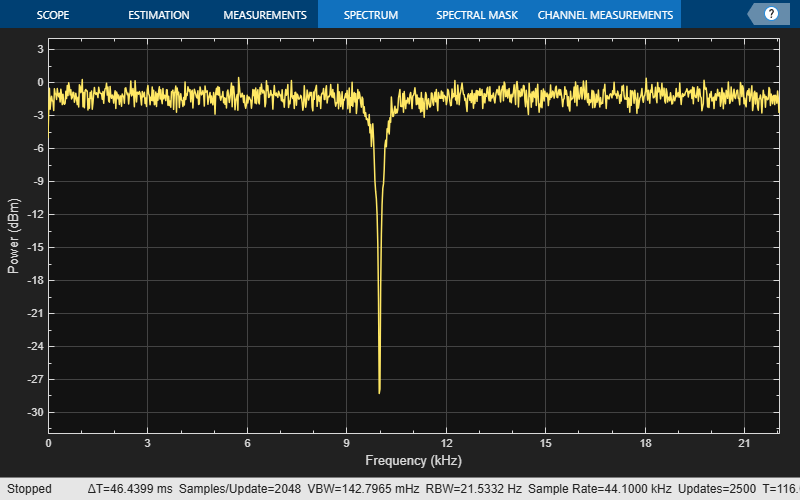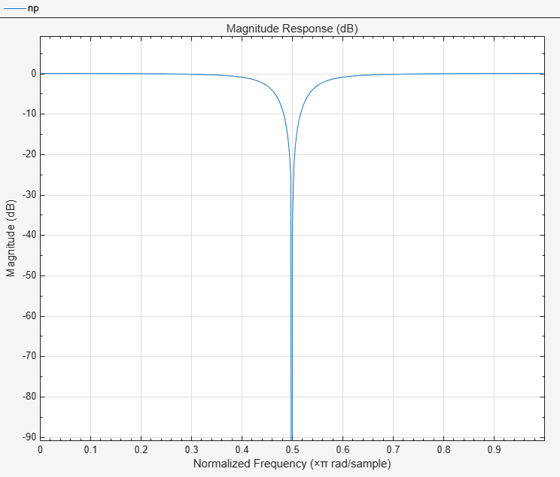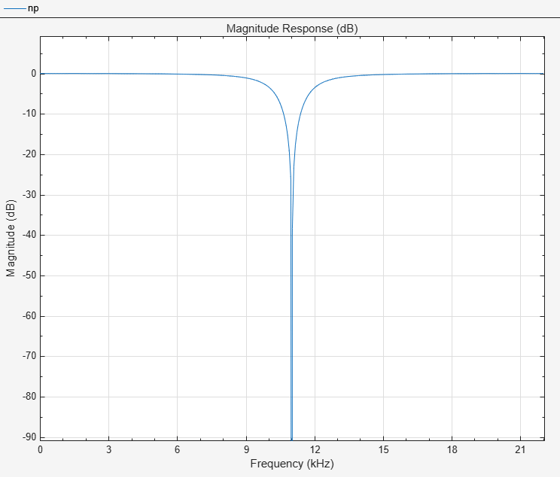# dsp.NotchPeakFilter

Second-order tunable notching and peaking IIR filter

## Description

The `NotchPeakFilter` object filters each channel of the input using IIR filter implementation.

To filter each channel of the input:

1. Create the `dsp.NotchPeakFilter` object and set its properties.

2. Call the object with arguments, as if it were a function.

## Creation

### Syntax

``npFilter = dsp.NotchPeakFilter``
``````npFilter = dsp.NotchPeakFilter('Specification','Quality factor and center frequency')``````
``npFilter = dsp.NotchPeakFilter('Specification','Coefficients')``
``npFilter = dsp.NotchPeakFilter(Name,Value)``

### Description

````npFilter = dsp.NotchPeakFilter` returns a second-order notching and peaking IIR filter that independently filters each channel of the input over time, using a specified center frequency and 3 dB bandwidth.```
``````npFilter = dsp.NotchPeakFilter('Specification','Quality factor and center frequency')``` specifies the quality factor (Q factor) of the notch or peak filter instead of the `3` dB bandwidth.```
````npFilter = dsp.NotchPeakFilter('Specification','Coefficients')` specifies the coefficient values that affect bandwidth and center frequency directly, rather than specifying the design parameters in Hz. This removes the trigonometry calculations involved when the properties are tuned.```

example

````npFilter = dsp.NotchPeakFilter(Name,Value)` returns a notch filter with each specified property name set to the specified value. Unspecified properties have default values.```

## Properties

expand all

Unless otherwise indicated, properties are nontunable, which means you cannot change their values after calling the object. Objects lock when you call them, and the `release` function unlocks them.

If a property is tunable, you can change its value at any time.

Set the specification as `'Bandwidth and center frequency'`, `'Quality factor and center frequency'`, or `'Coefficients'`.

Specify the filter’s `3` dB bandwidth as a finite positive numeric scalar in Hz. The value must be a scalar between 0 and half the sample rate.

Tunable: Yes

#### Dependencies

This property is applicable only if `Specification` is `'Bandwidth and center frequency'`.

Data Types: `single` | `double`

Specify the filter’s center frequency (for both the notch and the peak) as a finite positive numeric scalar in Hz. The value must be a scalar between 0 and half the sample rate.

Tunable: Yes

#### Dependencies

This property is applicable only if `Specification` is set to `'Bandwidth and center frequency'` or ```'Quality factor and center frequency'```.

Data Types: `single` | `double`

Specify the quality factor (Q factor) for both the notch and the peak filters. The Q factor is defined as the center frequency divided by the bandwidth. A higher Q factor corresponds to a narrower notch or peak. The Q factor should be a scalar value greater than 0.

Tunable: Yes

#### Dependencies

This property is applicable only if `Specification` is set to `'Quality factor and center frequency'`.

Data Types: `single` | `double` | `int8` | `int16` | `int32` | `int64` | `uint8` | `uint16` | `uint32` | `uint64` | `fi`

Specify the sample rate of the input in Hz as a finite numeric scalar.

Data Types: `single` | `double`

Specify the value that determines the filter’s `3` dB bandwidth as a finite numeric scalar in the range `[-1 1]`. The value `-1` corresponds to the maximum `3` dB bandwidth (`SampleRate`/4), and `1` corresponds to the minimum `3` dB bandwidth (0 Hz, an allpass filter).

Tunable: Yes

#### Dependencies

This property is only applicable if `Specification` is set to `'Coefficients'`.

Data Types: `single` | `double`

Specify the coefficient that determines the filter’s center frequency as a finite numeric scalar in the range `[-1 1]`. The value `-1` corresponds to the minimum center frequency (0 Hz), and 1 corresponds to the maximum center frequency (`SampleRate/2` Hz). The default is 0, which corresponds to `SampleRate/4` Hz.

Tunable: Yes

#### Dependencies

This property is only applicable if `Specification` is set to `'Coefficients'`.

Data Types: `single` | `double`

## Usage

### Syntax

``Y = npFilter(x)``
``[Yn,Yp] = npFilter(x)``

### Description

````Y = npFilter(x)` filters each channel (column) of the input signal, `x`, to produce the notch filter output, `Y`.```
````[Yn,Yp] = npFilter(x)` filters each channel of the input signal, `x`, to produce the notch filter output, `Yn`, and peak filter output, `Yp`.```

### Input Arguments

expand all

Input signal, specified as a vector or a matrix.

Data Types: `single` | `double`
Complex Number Support: Yes

### Output Arguments

expand all

Notch filter output, returned as a vector or a matrix.

Data Types: `single` | `double`
Complex Number Support: Yes

Peak filter output, returned as a vector or a matrix.

Data Types: `single` | `double`
Complex Number Support: Yes

## Object Functions

To use an object function, specify the System object™ as the first input argument. For example, to release system resources of a System object named `obj`, use this syntax:

`release(obj)`

expand all

 `getBandwidth` Get 3 dB bandwidth `getCenterFrequency` Get center frequency `getOctaveBandwidth` Bandwidth in number of octaves `getQualityFactor` Get quality factor `tf` Transfer function
 `step` Run System object algorithm `release` Release resources and allow changes to System object property values and input characteristics `reset` Reset internal states of System object

## Examples

collapse all

This example shows how to use dsp.NotchPeakFilter as a notch filter with center frequency of 5000 Hz and a 3 dB bandwidth of 500 Hz.

```npFilter = dsp.NotchPeakFilter('CenterFrequency',5000,'Bandwidth',500); sa = spectrumAnalyzer('SampleRate',44100,'AveragingMethod','exponential',... 'ForgettingFactor',0.96,'PlotAsTwoSidedSpectrum',false); for i=1:5000 y = npFilter(randn(1024,1)); sa(y); if (i==2500) % Tune center frequency to 10000 npFilter.CenterFrequency = 10000; end end release(npFilter) release(sa)```Create a `dsp.NotchPeakFilter` object with the `Specification` property set to `'Quality factor and center frequency'`. The default quality factor Q is 5, and the center frequency Fc is 11,025 Hz.

```np = dsp.NotchPeakFilter('Specification',... 'Quality factor and center frequency')```
```np = dsp.NotchPeakFilter with properties: Specification: 'Quality factor and center frequency' QualityFactor: 5 CenterFrequency: 11025 SampleRate: 44100 ```

Compute the 3 dB bandwidth of the notch peak filter using the `getBandwidth` function. The bandwidth is computed as the ratio of the center frequency and the quality factor, $\frac{\mathrm{Fc}}{\mathit{Q}}$.

`getBandwidth(np)`
```ans = 2205 ```

Visualize the filter response using `fvtool`.

`fvtool(np)`Create a `dsp.NotchPeakFilter` object with the `Specification` property set to `'Coefficients'`.

`np = dsp.NotchPeakFilter('Specification','Coefficients')`
```np = dsp.NotchPeakFilter with properties: Specification: 'Coefficients' BandwidthCoefficient: 0.7265 CenterFrequencyCoefficient: 0 SampleRate: 44100 ```

Determine the center frequency of the notch peak filter using the `getCenterFrequency` function. When the `Specification` is set to `'Coefficients'`, the center frequency is determined from the `CenterFrequencyCoefficient` value and the sample rate.

`getCenterFrequency(np)`
```ans = 11025 ```

Visualize the filter response using `fvtool`.

`fvtool(np)`Create a `dsp.NotchPeakFilter` object in the default configuration.

`np = dsp.NotchPeakFilter`
```np = dsp.NotchPeakFilter with properties: Specification: 'Bandwidth and center frequency' Bandwidth: 2205 CenterFrequency: 11025 SampleRate: 44100 ```

Determine the octave bandwidth of the filter using the `getOctaveBandwidth` function.

`getOctaveBandwidth(np)`
```ans = 0.2881 ```

Visualize the filter response using `fvtool`.

`fvtool(np)`Create a `dsp.NotchPeakFilter` object in the default configuration, where the `Specification `property is set to `'Bandwidth and center frequency'`.

`np = dsp.NotchPeakFilter`
```np = dsp.NotchPeakFilter with properties: Specification: 'Bandwidth and center frequency' Bandwidth: 2205 CenterFrequency: 11025 SampleRate: 44100 ```

Determine the quality factor of the filter using the `getQualityFactor` function. The quality factor is given by the ratio of the center frequency to the bandwidth.

`getQualityFactor(np)`
```ans = 5 ```

Visualize the filter response using `fvtool`.

`fvtool(np)`Create a `dsp.NotchPeakFilter` System object™. Obtain the coefficients of the transfer function corresponding to the notch and peak filters.

```notchpeak = dsp.NotchPeakFilter; [Bnotch,Anotch,Bpeak,Apeak] = tf(notchpeak)```
```Bnotch = 1×3 0.8633 -0.0000 0.8633 ```
```Anotch = 1×3 1.0000 -0.0000 0.7265 ```
```Bpeak = 1×3 0.1367 0 -0.1367 ```
```Apeak = 1×3 1.0000 -0.0000 0.7265 ```

`Bnotch` and `Anotch` are the vectors of numerator and denominator coefficients for the equivalent transfer function corresponding to the notch filter. `Bpeak` and `Apeak` are the vectors of numerator and denominator coefficients for the equivalent transfer function corresponding to the peak filter.

## Algorithms

The design equation for the peak filter is:

`$H\left(z\right)=\left(1-b\right)\frac{1-{z}^{-2}}{1-2b\mathrm{cos}{w}_{0}{z}^{-1}+\left(2b-1\right){z}^{-2}}$`

The design equation for the notch filter is:

`$H\left(z\right)=b\frac{1-2\mathrm{cos}{w}_{0}{z}^{-1}+{z}^{-2}}{1-2b\mathrm{cos}{w}_{0}{z}^{-1}+\left(2b-1\right){z}^{-2}}$`

with

`$b=\frac{1}{1+\mathrm{tan}\left(\Delta w/2\right)}$`

where ω0 = 2πf0/fs is the center frequency in radians/sample (f0 is the center frequency in Hz and fs is the sampling frequency in Hz). Δω = 2πΔf/fs is the 3 dB bandwidth in radians/sample (Δf is the 3 dB bandwidth in Hz). Note that the two filters are complementary:

The filter is implemented as follows:where

`$\begin{array}{l}{G}_{\text{3dB}}={a}_{2}=2b-1\\ {G}_{\text{cf}}=\frac{{a}_{1}-{a}_{1}{a}_{2}}{1-{a}_{2}{}^{2}}=-\mathrm{cos}{w}_{0}\end{array}$`

Notice that Gcf depends only on the center frequency, and G3dB depends only on the 3 dB bandwidth.

 Orfanidis, Sophocles J. Introduction to Signal Processing. Upper Saddle River, NJ: Prentice-Hall, 1996.